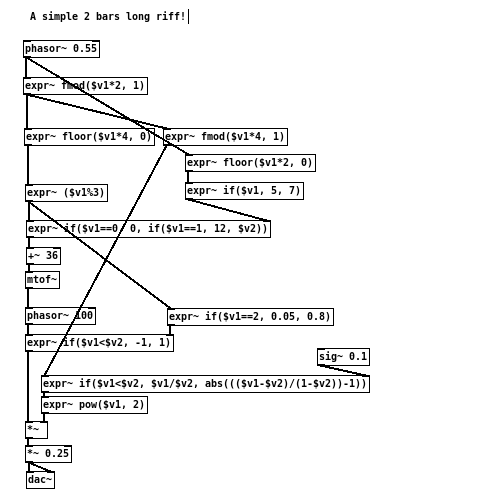```                  ______
/      |       ________
/       |____  /        \
/        |    \/   _      \
\       ___       /_\      \
\      \_/          .      \
\          /\_____/       /
\________/      |       /
|______/

```

# [THE_EXPR_METHOD] 005 Serial and parallel

20160118

Modulation, sequencing, division of time unit are simple ways to utilise a (Sawtooth) signal in order to achieve certain functions. How in which they are put together therefore determines the “arrangement” of the composition.

One simple approach in combining these elementary components, is to deduce the possibilities into two basic types: serial and parallel.

Serial, implies putting these functions one after another, so to produce desired outcome at the end of the chain. It typically results to control signals for modulation, or for logic control. The intermediate steps, in this case, involves resolving the correct timing frequency. In the example below, the fmod() is first used to divide the wave period of the incoming sawtooth, and then passed onto the next [expr~] to produce triangle wave.

```   [phasor~ ]->[expr~ fmod(\$v1*4, 1)]->[expr~ abs(abs(\$v1-0.5)-0.5)*2]
```

Parallel, on the other hand, refers to the diverging of signals, so that branches are created with each of the strand serving a different purpose. A common usage of this set-up would be to control various aspects of a given sound synthesis algorithm, from within a same time scope. For example, by using fmod() and floor() from the same timing signal, one can produce amplitude envelopes, as well as counters to alter the frequency of an oscillator at the same time. This process is very much the same as how control voltage (CV) is used in analog synthesiser.

```   [phasor~ ]->[expr~ fmod(\$v1*4, 1)]->[expr~ abs(abs(\$v1-0.5)-0.5)*2]->[send~ amp_env]
\ ->[expr~ floor(\$v1*4, 0)]->[expr~ if(\$v1%2, 2,1)]->[send~ freq]
```

As seen in above examples, serial connections are generally not very long, unless multiple steps are taken to derive the necessary timing. By grouping multiple serial process, a parallel arrangement is thus formed. Moreover, different group of parallel process can also be interconnected in a serial fashion, so to manipulate the composition at different scope of time.

In short, such a generalisation are guide lines in which can be used as a starting point when applying these techniques in composition. One other useful way in approaching the subject would be seeing it essentially the same as how CV signals are used analogue modular synthesiser.Get the source here

Exercise:

• Using the example patch, extend it into a 4 bar phrase.
• Using the example patch, make other voice/concurrent parts in the arrangement.